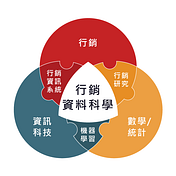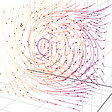# 一、常態/非常態分佈檢查

`ad1_list = data[data['廣告代號all'] == 'critei']['利潤'].tolist()ad2_list = data[data['廣告代號all'] == 'KDP']['利潤'].tolist()ad3_list = data[data['廣告代號all'] == '自然流量']['利潤'].tolist()`
`import scipy.statsscipy.stats.shapiro(ad1_list)scipy.stats.shapiro(ad2_list)scipy.stats.shapiro(ad3_list)`
`ShapiroResult(statistic=0.9818183183670044,pvalue=1.2465230236902158e-11)ShapiroResult(statistic=0.9621795415878296,pvalue=3.1705360319920887e-21)ShapiroResult(statistic=0.9822685122489929,pvalue=2.279967656292392e-21)`

# 二、非常態分佈

`# 進行Kruskal-Wallis testfrom scipy.stats.mstats import kruskalwalliskruskalwallis(ad1_list, ad2_list, ad3_list)`
`KruskalResult(statistic=4.584369300454998,pvalue=0.10104547153612356)`

# 二、視覺化分析結果

`import plotly.offline as pyimport plotly.graph_objects as gofrom numpy import meanfig = go.Figure()fig.add_trace(go.Scatter(              x= ['critei','KDP','自然流量'],              y= [mean(ad1_list), mean(ad2_list), mean(ad3_list)],              mode="lines+markers",              textfont=dict(              family="sans serif",              size=16,              color="royalblue"),               line=dict(color='royalblue', width=2),              ))`
`fig.update_layout(      title={          'text': "<b>廣告效益分析</b>",          'y':0.95,          'x':0.5,          'xanchor': 'center',},      yaxis_title='平均消費金額',      xaxis={          'title': '廣告',          'tickmode': 'linear'          },      width=1800,      height=960,      font=dict(          family="Courier New, monospace",          size=20,          color="lightslategrey"      )      )`
`# 另存互動式網頁檔案py.plot(fig, filename='無母數檢定－廣告效益分析', auto_open=True)# 另存圖檔fig.write_image("C:/Users/user/Desktop/無母數檢定1.1/無母數檢定－廣告益分析.png")`

--

--

--

## More from 行銷資料科學

Love podcasts or audiobooks? Learn on the go with our new app.## Applications of Computational Geometry## Fluid flow in food industry## My incredible journey with Street Group.## Virtual event analytics: how to use data to drive leads and sales